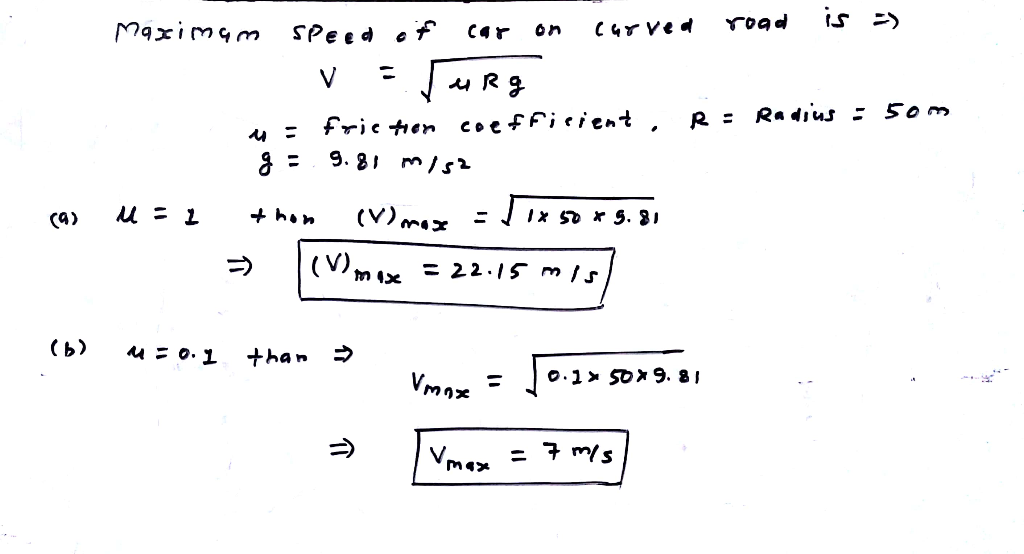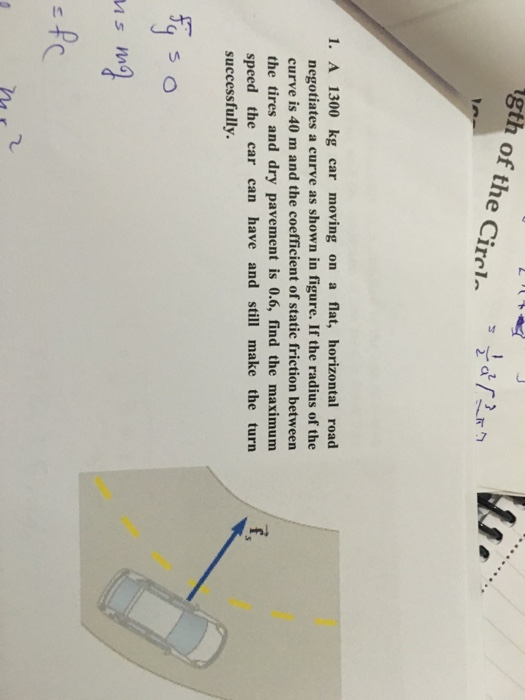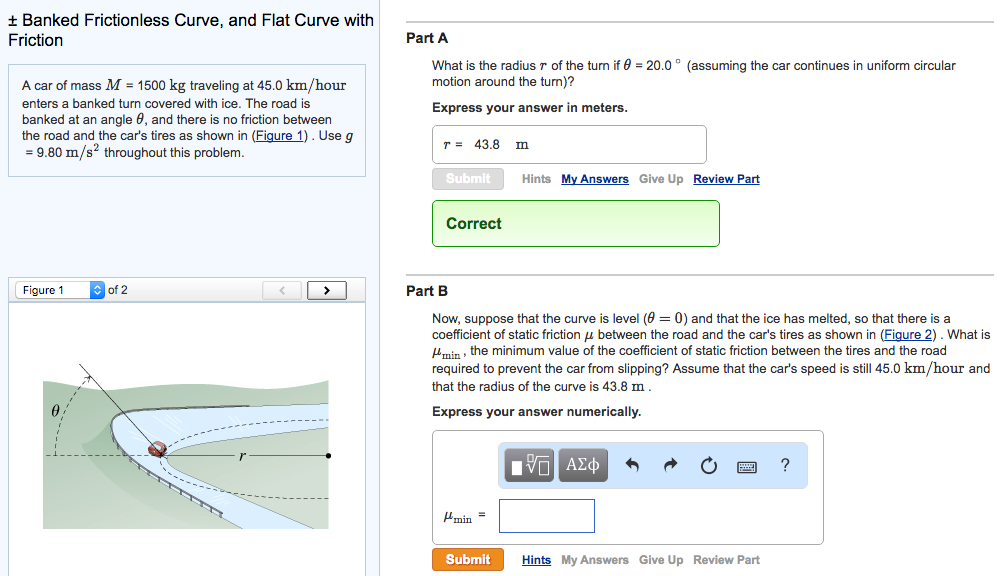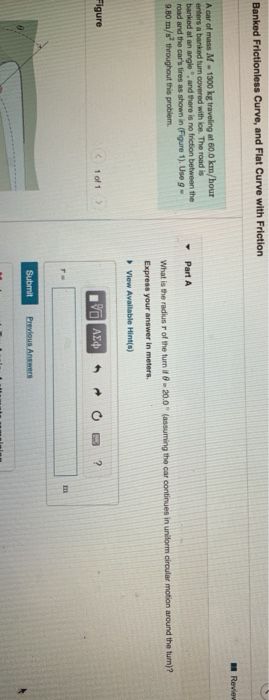Question

# You are driving your car along a flat, curved road; the curve in the road is...

You are driving your car along a flat, curved road; the curve in the road is a segment of a circle with radius 50 meters. (We call this a "radius of curvature"). How fast can the car drive around the curve if the coefficient of static friction between the tires and the road is 1.0 (tires on dry pavement)? What if the coefficient of friction is 0.2 (tires on ice)?#### Earn Coins

Coins can be redeemed for fabulous gifts.

Similar Homework Help Questions
• ### A car is driving around a flat highway curve that has a radius of 100 meters....

A car is driving around a flat highway curve that has a radius of 100 meters. The coefficient of friction between the wheels and pavement is 0.8. Please show work! A) Draw a force diagram for the car. What is the force responsible for the centripetal acceleration of the car? B) What is the fastest speed the car can drive around the curve?

• ### gth of the Cirrl 1. A 1300 kg car moving on a flat, horizontal road negotiates...gth of the Cirrl 1. A 1300 kg car moving on a flat, horizontal road negotiates a curve as shown in figure. If the radius of the curve is 40 m and the coefficient of static friction between the tires and dry pavement is 0.6, find the maximum speed the car can have and still make the turn successfully.

• ### PLEASE ANSWER PART B. THANKS! t Banked Frictionless Curve, and Flat Curve with Friction A car...PLEASE ANSWER PART B. THANKS! t Banked Frictionless Curve, and Flat Curve with Friction A car of mass M 1500 kg traveling at 45.0 km/hour enters a banked turn covered with ice. The road is banked at an angle 6, and there is no friction between the road and the car's tires as shown in (Figure 1). Use g 9.80 m/s2 throughout this problem. of 2 Figure 1 Part A What is the radius r of the turn if 0...

• ### 1000 kg car rounds a curve on a flat road of radius 20 m. if the...

1000 kg car rounds a curve on a flat road of radius 20 m. if the force of friction between dry pavement and tire is 5800 N, what is the maximum speed the car can safely make the turn?

• ### A car is moving at 16 m/s along a curve on a horizontal plane with radius...

A car is moving at 16 m/s along a curve on a horizontal plane with radius of curvature 49 m . The acceleration of gravity is 9.8 . What is the required minimum coefficient of static friction between the road and the car’s tires to keep the car from skidding?

• ### A 900 kg car passes a bend on a flat road with a radius of 60...

A 900 kg car passes a bend on a flat road with a radius of 60 m at a rate of 60 km / hour. Will the car be able to pass that bend, or will it slip if: (a) the road is dry and the coefficient of static friction is μS = 0.65; (b) ice road and μS = 0.3?

• ### Two cars are driving around a banked curve at a speed that is not too fast....

Two cars are driving around a banked curve at a speed that is not too fast. One car has really good tires (has lots of traction) and the other car has bad tires (bald with little traction).  Which of these cars is more likely to slide on the pavement as it goes around the curve? Two cars are driving around a banked curve at a speed that is not too fast. One car has really good tires (has lots of traction)...

• ### A flat (unbanked) curve on a highway has a radius of 240 m . A car...

A flat (unbanked) curve on a highway has a radius of 240 m . A car successfully rounds the curve at a speed of 37 m/s but is on the verge of skidding out. Part A If the coefficient of static friction between the car’s tires and the road surface were reduced by a factor of 2, with what maximum speed could the car round the curve? Express your answer in meters per second to two significant figures. part B...

• ### Banked Frictionless Curve, and Flat Curve with Friction Reviev A car of mass M - 1300...Banked Frictionless Curve, and Flat Curve with Friction Reviev A car of mass M - 1300 kg traveling at 60.0 km/hour enfers a banked turn covered with ice. The road is banked at an angle and there is no friction between the road and the car's tires as shown in Figure 1). Use 9 - 9.B0 m/s throughout this problem. Part A What is the radius of the turn it 8 - 20.0 (assuming the car continues in uniform circular...

• ### To test the performance of its tires, a car travels along a perfectly flat (no banking)...

To test the performance of its tires, a car travels along a perfectly flat (no banking) circular track of radius 101 m. The car increases its speed at uniform rate of 3.56 m/s^2 until the tires start to skid. If the tires start to skid when the car reaches a speed of 16.9 m/s what is the coefficient of static friction between the tires and the road?